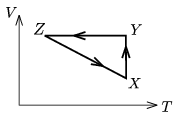Mathematical and Physical Journal
for High Schools
Issued by the MATFUND Foundation
 Already signed up? New to KöMaL?

#Problem P. 3899. (May 2006)

P. 3899. A sample of ideal gas is taken through the cyclic process shown in the figure. At state X TX=373 K, VX=5 dm3, and at state Z VZ=12 dm3, TZ=273 K. At which state of the process ZX will the pressure be the same as the pressure at state Y?(4 pont)

Deadline expired on June 12, 2006.

### Statistics:

 94 students sent a solution. 4 points: 80 students. 3 points: 3 students. 2 points: 5 students. 1 point: 2 students. Unfair, not evaluated: 4 solutionss.

Problems in Physics of KöMaL, May 2006